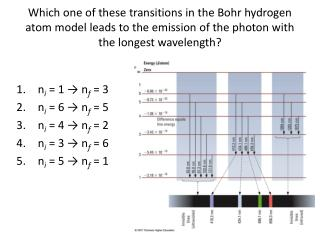DownloadDownload Presentationn i = 1 → n f = 3 n i = 6 → n f = 5 n i = 4 → n f = 2 n i = 3 → n f = 6

# n i = 1 → n f = 3 n i = 6 → n f = 5 n i = 4 → n f = 2 n i = 3 → n f = 6

Télécharger la présentation## n i = 1 → n f = 3 n i = 6 → n f = 5 n i = 4 → n f = 2 n i = 3 → n f = 6

- - - - - - - - - - - - - - - - - - - - - - - - - - - E N D - - - - - - - - - - - - - - - - - - - - - - - - - - -
##### Presentation Transcript

1. Which one of these transitions in the Bohr hydrogen atom model leads to the emission of the photon with the longest wavelength? • ni = 1 → nf = 3 • ni = 6 → nf = 5 • ni = 4 → nf = 2 • ni = 3 → nf = 6 • ni = 5 → nf = 1

2. Dental lasers are based on neodymium yttrium aluminum garnet (Nd-YAG) crystal, with an emission wavelength of 1066 nm. What is the frequency of this laser? • 2.812 × 1014 Hz • 2.812 × 105 Hz • 3.195 × 102 Hz • 3.196 × 1018 Hz

3. The nitrogen laser emits light at 337.1 nm. What is the energy of this light? • 6.696 × 10-32 J • 2.118 × 10-31 J • 5.893 × 10-20 J • 5.893 × 10-19 J

4. Which of the following is a list of allowed quantum numbers for an electron? • n = 1, l = 1, ml = 0, ms = ½ • n = 2, l = 0, ml = 1, ms = ½ • n = 3, l = 0, ml = 0, ms = ½ • n = 4, l = 3, ml = 4, ms = -½

5. What is the correct electron configuration for sulfur? • 1s22s22p63s13p5 • 1s22s22p63s23p4 • 1s22s22p73s23p2 • 3s23p4

6. What is the correct condensed electronic configuration for Fe3+? • [Ar]4s23d6 • [Ar]4s23d3 • [Ar]3d5 • [Ar]4s23d9

7. Which is the largest atom below? • O • N • Al • Sr

8. Key 1. 2 2. 1 3. 4 4. 3 5. 2 6. 3 7. 4